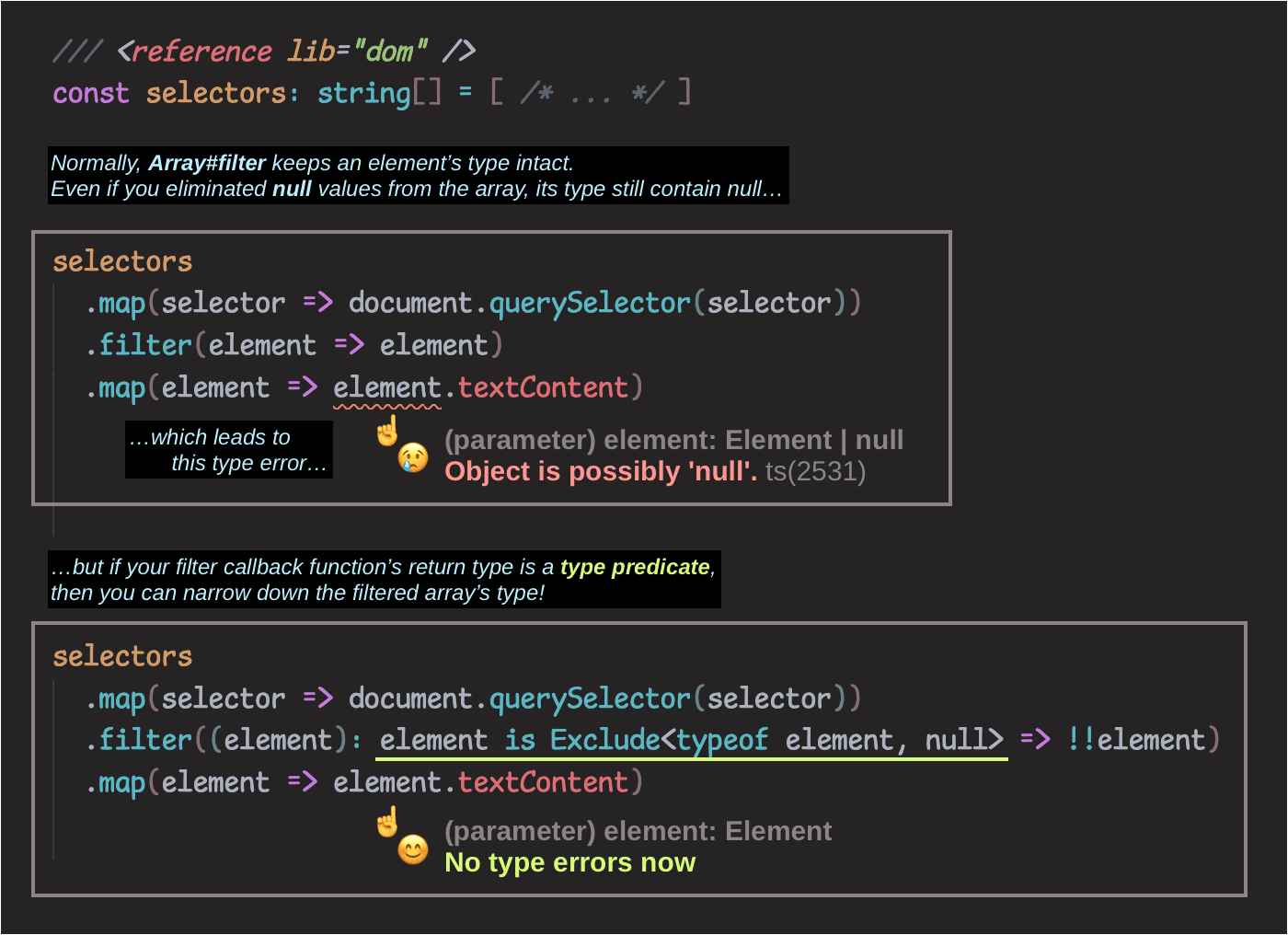Today(opens new window) I learned that in TypeScript, passing a function whose return type is a type predicate to `Array#filter` can narrow down its return type!I have an array where some elements may either be an object or null (`Array<T | null>`). Usually I do this:

``````array.filter(x => x)
``````

While null values are effectively removed in runtime, the type system still sees the type of the resulting array as `Array<T | null>`. I just learned that I can write it this way instead:

``````array.filter((x): x is T => !!x)
``````

…or if I don’t want to hardcode the type of the element:

``````array.filter((x): x is NonNullable<typeof x> => !!x)
``````

Now I get `Array<T>`.

Other techniques exist that doesn’t require type annotations, such as using `flatMap` instead.

``````array.flatMap(x => x ? [x] : [])
``````

Or using `_.compact` or its equivalent:

``````const compact = <T,>(array: readonly T[]) => array.filter((x): x is NonNullable<T> => !!x)
compact(array)
``````